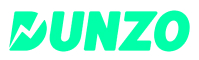New update is available. Click here to update.
Last Updated: 4 Mar, 2022

# Shortest Common Supersequence

Hard+8 more companies

## Problem statement#### Note: A string 's' is a subsequence of string 't' if deleting some number of characters from 't' (possibly 0) results in the string 's'.

##### For example:
``````Suppose ‘A’ = “brute”, and ‘B’ = “groot”

The shortest supersequence will be “bgruoote”. As shown below, it contains both ‘A’ and ‘B’ as subsequences.

A   A A     A A
b g r u o o t e
B B   B B B

It can be proved that the length of supersequence for this input cannot be less than 8. So the output will be bgruoote.
``````

#### Input Format:

``````The first line of the input contains a single integer ‘T’ representing the no. of test cases.

The first line of each test case contains a single string ‘A’, denoting the first string described in the problem.

The second line of each test case contains a single string, ‘B’, denoting the second string described in the problem.
``````

#### Output Format:

``````For each test case, print the shortest string ‘S’, which contains ‘A’ and ‘B’ as its subsequences.

Print a separate line for each test case.
``````
##### Note:
``````You are not required to print anything; it has already been taken care of. Just implement the function and return the answer.
``````

#### Constraints:

``````1 ≤ T ≤ 100
1 ≤ |A|, |B| ≤ 1000
Both strings consist of only lowercase English letters.
1 ≤ Σ(|A|+|B|) ≤ 3000

Time limit: 1 Sec
``````## Approaches

### 01 ApproachLet’s consider two substrings of length m and n, respectively. Now we can have two situations:-

Both the strings end with the same element:

So for finding their shortest common supersequence, what we have to do is we will shorten each string by removing the last element. Then we will find the shortest common supersequence of shortened lines, and then the removed element will be appended to the shortest common supersequence.

SCS(A[1…..m], B[1…...n]) = SCS(A[1….m-1], B[1...n-1]) + A[m]

Suppose two given strings are not ending at the same character:

In this situation the shortest common supersequence will be the shorter of the two sequences SCS(A[1...m-1], B[1..n]) + A[m] and SCS(A[1...m], B[1...n-1]) + B[n].

#### Algorithm:

Function shortestSupersequenceRec

Function arguments - string ‘A’, string ‘B’, integer ‘N’ representing the length of A’s prefix to be considered, integer ‘M’ representing the length of B’s prefix to be considered.
Returns - Shortest supersequence of A[1,2,...N] and B[1,2,...M].

• If n == 0
• Return prefix of B of length m
• If m == 0
• Return prefix of A of length n
• If a[n-1] == b[n-1]
• Return shortestSupersequenceRec(a, b, n-1, m-1) + a[n-1]
• Declare and initialize string sa = shortestSupersequenceRec(a, b, n-1, m) + a[n-1]
• Declare and initialize string sb = shortestSupersequenceRec(a, b, n, m-1) + b[m-1]
• Return the shorter string out of sa and sb

Given Function

• Declare and initialize integers n = length of A and m = length of B
• Return shortestSupersequenceRec(a, b, n, m)